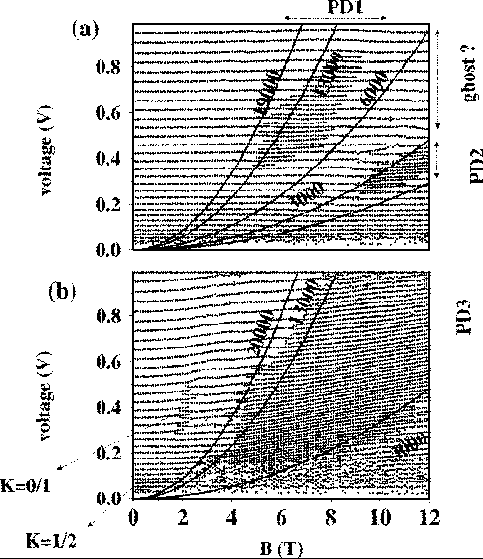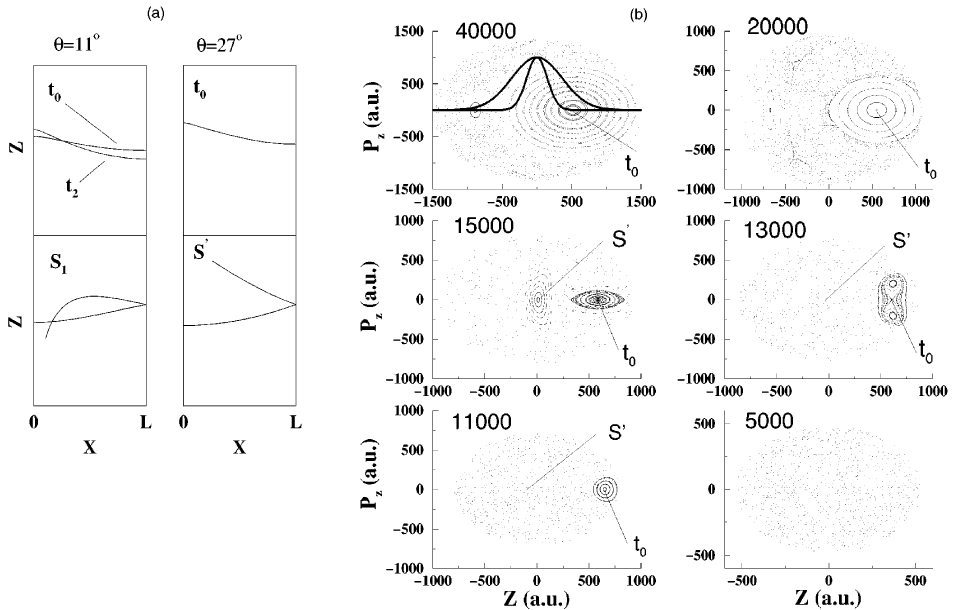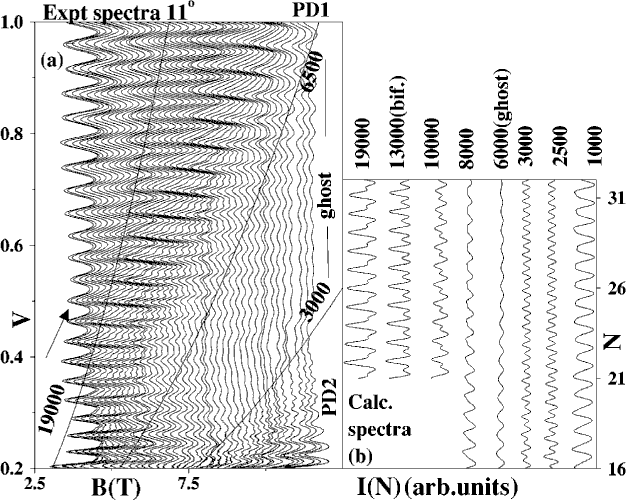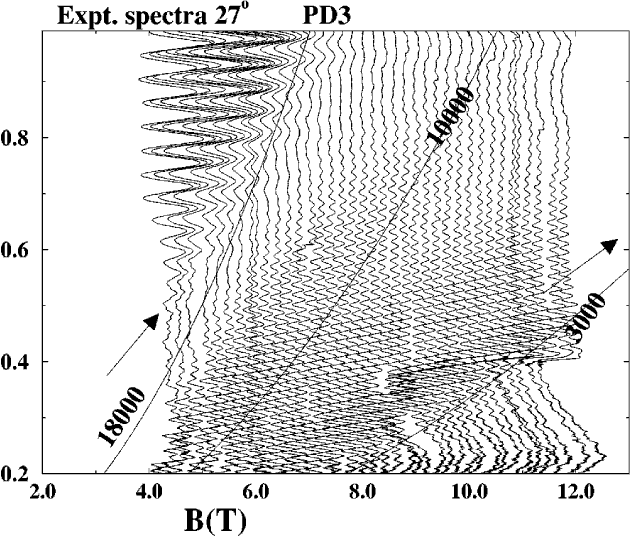Quantum wells in tilted fields: Semiclassical analysis and experimental evidence for effects "beyond" periodic orbits
D. S. Saraga and T. S. Monteiro
Physical Review E (Statistical Physics, Plasmas, Fluids, and Related Interdisciplinary Topics) -- May 1998 -- Volume 57, Issue 5 pp. 5252-5265
AbstractExperimental data plotting the positions of maxima in the I(V) traces as a function of magnetic field B. The curves are parabolas of constant V/B2 , which correspond approximately to constant classical dynamics. They are labeled with the value of e = F/B2 . PD1, PD2, and PD3 denote period-doubling regions. The features associated with the changeover of the quantum number of the torus series with the strongest current amplitude are indicated by the torus K quantum numbers. q = 11°. q = 27°.(a) Main periodic orbits which domi-nate the current oscillations at q = 11° and q = 27°. t0 gives the main period-one current. t2 is an unstable PO, which coalesces with t0 in a series of tangent bifurcations. S1 1 and S' are 3-bounce orbits responsible for some of the period-doubling regions. (b) Poincare surfaces of section for q = 27° for different values of e = F/B2 . The dynamics becomes progressively more chaotic as e decreases.Comparison between experimental and theoretical line profiles at q = 11 o showing effects of approximate scaling dynamics. (a) Experiment: set of I-V traces (reduced current) showing characteristic line profiles roughly along parabolas of constant e = F/B2. . The period-doubling regions PD1 and PD2 are shown along with the ghost region where the results are not due to a real PO. The arrows indicate the semi-classical limit. (b) Theory: smoothed theoretical spectra. The quantum number N is proportional to magnetic field B. The range N=12-42 corresponds to voltage range 0.121.1 V. With decreasing e, right asymmetric profiles ( ~ 19 000) evolve into symmetric perioddoubled profiles at the bifurcation. Below the tangent bifurcation ( ~ 6500) the ghost has a weak sinusoidal profile. PD2 is mainly due to S1 but has substantial period one interference, indicating that t0 is still accessible.Experimental traces at q = 27° showing the broad plateau of period-doubled current corresponding closely to the range of the quantum maximum rather than the range of the semiclassical current. This indicates that contribution is not due to real PO over about half the experimental period-doubled range (below e ~ 8000 and above e ~ 18 000).